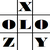anything in Excel...

It's Excel-o-lozy...

### In Excel Dig or Extract only Numbers or only Alphabets from Alphanumeric value by formula.. under formulaFix by Excelolozy.

www.excelolozy.com - To Dig out Number from an AlphaNumeric text, where no space or any special character is there in between, is a tough task...

Right???

Wrong...

Problem is just that "Text to Columns" option doesn't work in this case being no separator in between.

BUT nothing tough as far as Excel is concerned... ;)

"Dig" is a Formula that can extract not only Numbers but even Alphabets only from any AlphaNumeric text.

More over the formula can also extract Alphabets as follows :

• e.g. to extract Numeric value from "123ABC", following is the solution :
=Dig("123ABC")
result of which is shown as "123".

• e.g. to extract Numeric value from "1A2B3C", following is the solution :
=Dig("1A2B3C")
result of which is shown as "123".

More over the formula can also extract Alphabets as follows :

• e.g. to extract Alphabets from "123ABC", following is the solution :
=Dig("123ABC")
result of which is shown as "ABC"

• e.g. to extract Alphabets from "1A2B3C", following is the solution :
=Dig("1A2B3C")
result of which is shown as "ABC".
The formula "Dig" is available within tool named "formulaFix".

"formulaFix" is a "Formula Fixing Tool" that provides various formulas that dont exist in Excel.